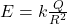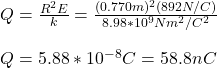## The electric field everywhere on the surface of a thin, spherical shell of radius 0.770 m is of magnitude 892 N/C and points radially toward

Question

The electric field everywhere on the surface of a thin, spherical shell of radius 0.770 m is of magnitude 892 N/C and points radially toward the center of the sphere. (a) What is the net charge within the sphere’s surface

in progress 0
4 weeks 2021-08-18T11:09:43+00:00 1 Answers 0 views 0

Q = 58.8 nC

Explanation:

For distance just above the surface of the spherical shell and higher, electric field of the shell can be considered as the electric field generated by a charge Q at the center of the spherical shell:

To calculate the net charge within the sphere’s surface you use the following formula:(1)

Q: net charge of the spherical shell

R: radius of the sphere = 0.770m

E: magnitude of the electric field for points just above the sphere’s surface = 892N/C

k: Coulomb’s constant = 8.98*10^9 Nm^2/C^2

You solve the equation (1) for Q and replace the values of the other parameters:The net charge within the sphere’s surface is 58.8nC